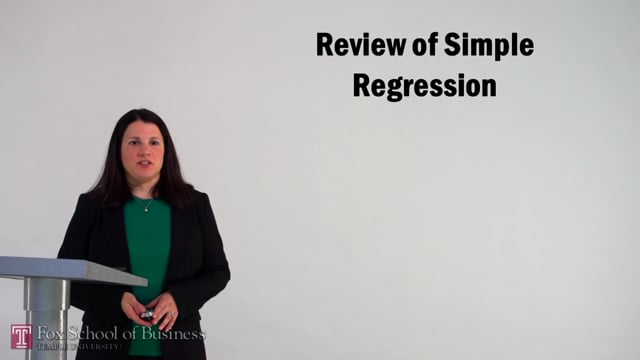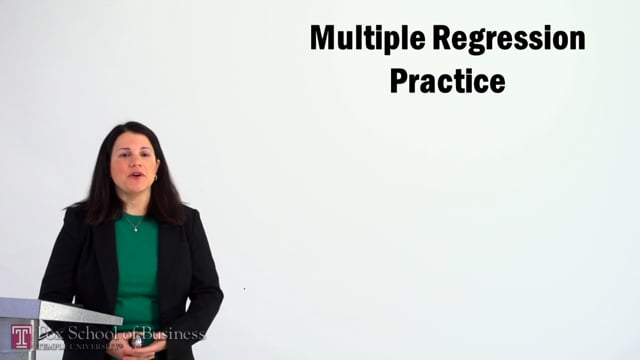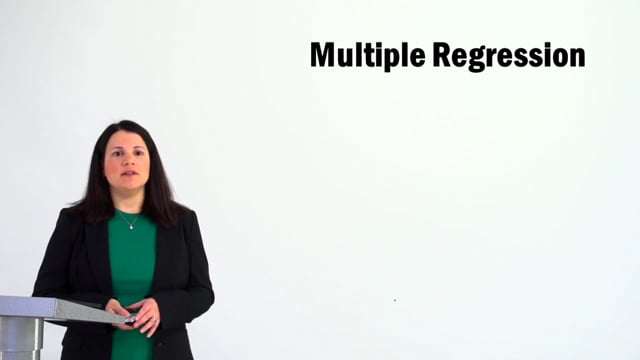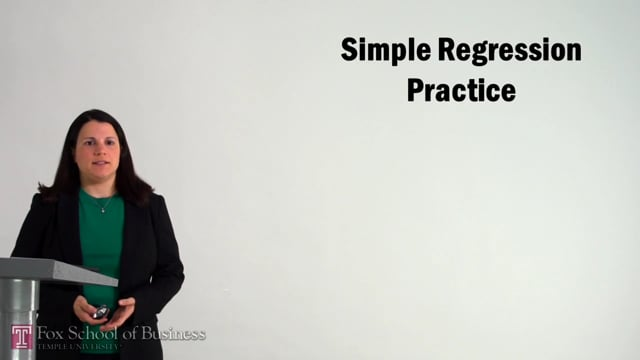COVID-19 Information: The latest about how Temple is safeguarding our community.

Support / FAQs

Linear Regression ModelReview of Simple Regression

Total Running Time: 27:40
Review of Simple RegressionMultiple Regression Practice

Total Running Time: 37:02
Multiple Regression PracticeMultiple Regression

Total Running Time: 31:32
Multiple RegressionSimple Regression Practice

Total Running Time: 23:28
Simple Regression Practicevault.temple.edu